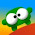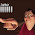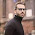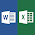## Wednesday, August 26, 2009

### Distance to a Quadratic bezier curve

Recently, after viewing this illuminating animated GIFs, I realized that finding the closest point on a quadratic Bezier curve to a given point M might be not so difficult...and very useful for detecting collision between a disk and a quadratic curve.

Quadratic Bezier curves are familiar to flash programmers, because these are the curves they draw with functions like Movieclip.moveTo() and Movieclip.curveTo().

Such Bezier curve is defined by 3 points: P0, P1, P2.
P0 and P1 are the extreme points, and P1 is a middle point determining curvature.

The curve can be parametrized with the formula:

P(t) = (1-t)²P0 + 2t(1-t)P1 +t²P2.
t is a parameter variable walking from 0 to 1. When t = 0, you are at point P0, and when t = 1, u're at P2.Each point of the curve has a corresponding "t".
The above-mentioned animated GIFs greatly help to "geometrically" grasp not only the quadratic curves, but also higher order Bezier curves.

Now, the key step is to get the derivative (back to school!!) of P(t):
dP/dt(t) = -2(1-t)P0 + 2(1-2t)P1 + 2tP2
which can be rewritten as:
dP/dt(t) = 2(A+Bt)
where A = (P1-P0) and B = (P2-P1-A)
(note: A and B have a geometric signification: they are the diagonals of the parallelogram defined by P0,P1,P2 and a fourth point kind of "opposite" from P1)

The derivative is a vector representing the "speed" at point defined by P(t). It also has the property to be a tangent to the curve at point P(t).

Now let M(x,y) be our point (which can be anywhere) and we want to find out a point P' (defined by t') on Bezier curve that is the closest possible to M.

P(t') will meet the condition:

MP.dP/dt = 0
(where "." is the dot product of 2 vectors)
that is, MP will be orthogonal to the tangent at P.
Rewritting this condition yields:
(M - (1-t)²P0 + 2t(1-t)P1 +t²P2).(A+Bt) = 0

...After a (boring) calculus you get a third degree equation (ouch!!):
at3 + bt² + ct + d = 0,
where a = B², b = 3A.B, c = 2A²+M'.B, d = M'.A, (and M' = P0-M)

If we can solve this equation, we get 3 possible solutions for t !!
How to solve a third degree equation is explained here (in french).
Now we have a maximum of 3 solutions t0, t1, t2 and find the one fitting in [0,1] and minimizing dist(M, P(t)) is easy !!Here is a demo:

Drag the circled dots
P0 and P2 are the blue points, P1 is green , M is red.
T
he yellow dot is the closest point, the orange dot indicates the normal to M.

After this, I realized that Andre Michelle had already posted a ball collision detection on his blog a long time ago. But the source wasn't available (at least I didn't work for nothing)...

The source code (AS2, FLA CS3 + Bezier.as).
Translating it into as3 should be no problem.

[UPDATE 2012/08/06] I fixed the issue pointed by makc3d. Now the end points are included as a possible solution, which is more consistent. I added a flad "onCurve" indicating if the closest point is on the curve itself (true), or one of the end points (false). The "oriented distance" is negative when the point is on the right of the path formed by [P0,P1,P2]. For backward compatibility, I kept this info in the variable "orientedDist", but it loses its meaning when the closest point is not on the curve.

1.seems like you didnt trim your solution to actual segment

2.Mh.. do you mean that I don't check the solution for t are in [0,1] interval?.. I just checked my code and it's done at line 137:
if (t>=0 && t<=1)
Then, I don't get it.. Can you send me (olive_at_gludion.com) a screenshot showing the problem?

3.oh got it.. you probably suggest I should mention it in the post. Now it's edited. Thanks ;)

4.no I mean that at certain positions dots just disappear, when they should remain at the segment endpoints.

5.yes, the dots disappear if no point P of the bezier curve meets the condition MP.dP/dt = 0.
In this case, the function returns null.
In my game, it usually corresponds to a case of point M colliding another curve.

But actually you're right, it should provide one of the endpoints in such cases.

It's easy to add some code testing which one of the 2 endPoints is closest when the result is null.

6.Those wikipedia gifs are indeed awesome. Thanks for your post!

7.BTW, a complete solution should always check distance to both endpoints (whether or not the result is null) as there are certain cases where this returns the wrong distance because there are a few corner cases in the initial premise - the shortest distance is always tangent to the curve.

See this image for an incorrect response from this function:

http://www.onetacoshort.com/trash/bez_dist.jpg

As you said, if there were always other curves, this this wouldn't be a problem. But with only this curve in consideration, distance is reported as -237, but it's actually closer to the left endpoint.

Also, please don't take my comments as critical - I really appreciate your effort and just want it to be more complete. =) Cheers and great work!

8.wow !!

thx a lot for your input :D

9.Thanks very much for this post.
Aways great to find complex things simplified.
:)

10.Thank you for posting this! I had some strange results with the code though. So I did the same for a cubic bezier and it work perfectly, giving a 5th degree polynomial.

I then did the calculus for the quadratic bezier myself and came up with a different solution, I think...

Setting A = P1 - P0 and B = P2 - P1 - A

I got a = B.2*(2P1 - P0 - P2) . is the dot product
b = B.4*(P0 - P1) + A.2*(2P1 - P0 - P2)
c = B.2*(M - P0) + A.4*(P0 - P1)
d = A.2*(M - P0)

this differ some to your solution. It works for me, just thought I should post it here.

Thanks!

/Per

11.Hello, thanks for sharing!

However, in your formula (a = B.2*(2P1 - P0 - P2)), it's actually equivalent to:
a = -2*B.B, because B = P2-2P1+P0.

So, I think both our solutions are about the same (probably all your a,b,c,d are multiplied by -2 or 2, which doesn't change the validity of the equation).
In my formula, the purpose of A and B was precisely to simplify the expression of a,b,c,d and avoid any reference to P0,P1,P2.

12.Thanks! I suspected it was the same, but I didn't check it. I must have put a sign wrong somewhere in my code. BTW do you know how the formula looks to check if two quadratic bezier's intersect? Or if they don't the points on the curves that are closest to each other?

Thanks!

/Per

13.Sorry, I don't know..
But it seems that Google does ;-)

14.I want to know on which side the point lies.(side where distance is negative or side where distance is positive). Your negative distance is helpful to me. But curious to know how a distance is coming out negative.

15.great post , thanks :)

16.I updated the class and the demo as suggested by makc3d... sorry for the long delay !! ;)

17.I'm a little confused by your expression of "MP". This is the line segment from M to P(t'), right? You use the expanded expression:

(M - (1-t)²P0 + 2t(1-t)P1 +t²P2).(A+Bt) = 0

since this is supposed to be the vector M-P(t'), should that be:

(M - ((1-t)²P₀ + 2t(1-t)P₁ +t²P₂)) · (A+Bₜ) = 0

instead? The one that is currently on the page subtracts the P₀ term from M, but then adds the P₁ and P₂ terms, rather than subtracting the entire Bezier curve from M...

As for the math symbols, you can replace the . with the unicode center dot, ·, which is U+B7. It should work fine on any page that's set to utf-8 encoding. And the subscripts ₀, ₁, ₂, and ₜ can either be done using the "sub" element, or using the unicode letters (they have their own =D)

18.Thank you very much for posting this. It was exactly what I was looking for for a game I am working on.

19.There is somethink weird going on inside the quadratic (D=0) section. It look, it "snap" to the inflection point thus giving incorrect results. I rewrote your code to HLSL and this image is what I get: http://goo.gl/Ge1PE . Note that even this is special case (P0 == P2), its wrong anytime the angle is too sharp (in my test case up to P0[-80;0], P1[0;0] P2[-80; 50]). I still am not able to find out whats the problem. Have you guys any idea? Thanks!

20.Thank you so much! I've been trying to figure out how to do this for quite a while. It was driving me crazy!

21.This comment has been removed by the author.

22.Thanks for this post--it is helpful. However--there are some areas where the math gloss makes things a little hard to follow--specifically where you develop the at3 + bt² + ct + d = 0. Is there any sort of link to this derivation available? Thanks

23.Something related

24.Hi,
Whastapp +905327099681

25.I'm keen the multitude of blogposts, I just earnestly cherished, I'd like the informatioin needed for it again, as it's very marvelous., Cheers in support of conveying. distance calculator UK

26.It is important to discover distance calculator UK the distance between the two points on the guide.

27.A delightful reading for anyone who loves reading blogs. used cars on installments in lahore - standard chartered credit card

28.What a fabulous post this has been. Never seen this kind of useful post. I am grateful to you and expect more number of posts like these. Thank you very much. Petty Cash Receipt Template Excel

29.Very good article and thanks for sharing such information. Already Read your Recent Post its Great Thanks informative speech outline | bowling score sheet | printable reading log | to whom it may concern letters | memorandum of understanding